# SAT II Math I : Equations Based on Word Problems

## Example Questions

### Example Question #11 : How To Find The Amount Of Sales Tax

If Jane buys four cans of soda at $1.56 each and pays$6.93 total, what is the percentage of the sales tax?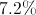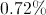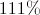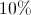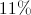Explanation:

# How to calculate the amount of sales tax?

1.      Convert tax percentage into a decimal by moving the decimal point two spaces to the left.
2.      Multiple the pre-tax value by the newly calculated decimal value in order to find the cost of the sales tax.
3.      Add the sales tax value to the pre-tax value to calculate the total cost.

In order to calculate the sales tax of an item, we need to first multiply the pre-tax cost of the item by the sales tax percentage after it has been converted into a decimal. Once the sales tax has been calculated it needs to be added to the pre-tax value in order to find the total cost of the item. Let's start by working with an example. If a magazine costs $2.35 and has a 6% sales tax, then what is the total cost of the item. First, we need to convert the sales tax percentage into a decimal by moving the point two spaces to the left.Now, we need to multiply the pre-tax cost of this item by this value in order to calculate the sales tax cost.Round to two decimal places since our total is in dollars and cents.Last, add this value to the pre-tax value of the item to find the total cost.## Calculating the sales tax percentage of a total: If we are given the total cost of an item or group of items and the pre-tax cost of the good(s), then we can calculate the sales tax percentage of the total cost. First, we need to subtract the pre-tax value from the total cost of the purchase. Next, we need to create a ratio of the sales tax to the pre-tax cost off the items. Last, we need to create a proportion where the pre-tax cost is related to 100% and solve for the percentage of the sales tax. Let's start by working through an example. If a person pays$245.64 for groceries that cost $220.00 pre tax, then what is the sales tax percentage for the items. First, subtract the pre-tax value from the total cost of the items to find the sales tax cost.Next, create a ratio of the sales tax to the pre-tax cost of the items.Last, create a proportion where the pre-tax value is proportional to 100% and solve for the percentage of sales tax.Cross multiply and solve.Isolate the sales tax percentage to the left side of the equation by dividing each side by the pre-tax value.Round to two decimal places since our answer is in dollars and cents.Last, we can check this answer by calculating the sales tax percentage of the total as seen previously. First, we need to convert the sales tax percentage into a decimal by moving the point two spaces to the left.Now, we need to multiply the pre-tax cost of this item by this value in order to calculate the sales tax cost.Round to two decimal places since our total is in dollars and cents.Last, add this value to the pre-tax value of the item to find the total cost.Our answers check out; therefore they are correct. Now, let's use this information to solve the given problem. ## Solution: If each soda cost$1.56 and Jane bought four, the total for the sodas was: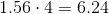And she paid \$6.93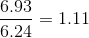The sales tax was 11%.

### Example Question #1 : Equations Based On Word Problems

Which of the following phrases can be written as the algebraic expression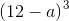?

Three times the difference of twelve and a number

Three times the difference of a number and twelve

The cube of the difference of a number and twelve

Twelve decreased by the cube of a number

The cube of the difference of twelve and a number

The cube of the difference of twelve and a number

Explanation:is the cube of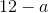, which is the difference of twelve and a number. Therefore,is "the cube of the difference of twelve and a number."

### Example Question #2 : Equations Based On Word Problems

If Bob fills a bucket with 9 logs that weigh 1.22 pounds each and the total weight of the bucket and logs 13.3 pounds, what percentage of the total weight is the bucket?

.1744%

17.44%

2.32%

18.60%

15.9%

17.44%

Explanation:

This question involves muliple steps to attain the correct answer.

To solve for the weight of the bucket (we will let 'x' represent this) the following equation can be set up: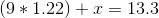To isolate x, subtract 9 * 1.22 (which is equal to 10.98) from both sides. We find x to be equal to 2.32.

The question asks us for the percentage of the total weight, so to find this, we must divide 2.32 by the total weight and multiple our answer by 100.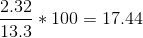Rounded to two decimal points of accuracy, the final answer is 17.44%.

### Example Question #3 : Equations Based On Word Problems

Which of the following phrases can be represented by the algebraic expression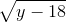?

The square root of a number multiplied by negative eighteen

The square root of the difference of a number and eighteen

The square root of the product of a number and negative eighteen

Eighteen less than the square root of a number

The square root of the difference of eighteen and a number

The square root of the difference of a number and eighteen

Explanation:is the square root of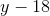,which is the difference of a number and eighteen; therefore,is "the square root of the difference of a number and eighteen".

### Example Question #4 : Equations Based On Word Problems

Which of the following phrases can be represented by the algebraic expression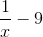?

The product of negative nine and the reciprocal of a number

Nine less than the reciprocal of a number

Nine decreased by the reciprocal of a number

The reciprocal of the product of negative nine and a number

The reciprocal of the difference of a number and nine

Nine less than the reciprocal of a number

Explanation:is nine less than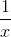, which is the reciprocal of a number. Therefore,is "nine less than the reciprocal of a number. "

### Example Question #5 : Equations Based On Word Problems

Which of the following phrases can be written as the algebraic expression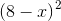?

The square of the difference of a number and eight

The difference of eight and the square of a number

The difference of eight and twice a number

The square of the difference of eight and a number

Twice the difference of eight and a number

The square of the difference of eight and a number

Explanation:is the square of the expression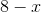is the difference of eight and a number.

Subsequently,is "the square of the difference of eight and a number".

### Example Question #2 : Equations Based On Word Problems

Jim sells apples for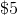per pound and oranges for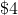per pound.  What is a formula to find therevenue forpounds of apples and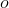pounds of oranges?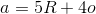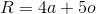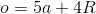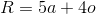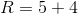Explanation:

Since you are solving for, that goes on one side of the equation.and's revenues are based on how much they sell for per pound.

So for every pound of apples they makedollars or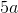.

For every pound of oranges they makedollars or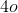.

To put it all together, you get.

### Example Question #7 : Equations Based On Word Problems

Sam currently has five ducks. He will receive 3 new ducks every two months. How many individual ducks must he have after 7 months?Explanation:

Sam starts out with five ducks. He receives 3 ducks after every two months.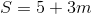where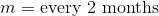.

After the first 2 months, he will have 8 ducks.

After the next 2 months, he will have 11 ducks.

After the next 2 months, he will then have 14 ducks.

However, Sam will not receive 3 ducks until the 8th month. This means that he will only have 14 ducks in possession by the 7th month.

The answer is: